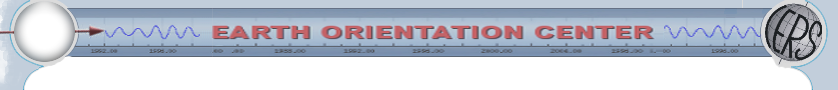BACK
HOME SHOW THIS PAGE
POLAR MOTION
 COORDINATES OF THE POLE - POLAR MOTION Low frequency polar motion The motion of the rotation axis of the Earth relative to the crust is represented by the Earth Orientation Parameter x and y. In the terrestrial frame, the equatorial coordinate of the pole are x and -y. It has two major components : (i) a free oscillation with period about 435 days (Chandler wobble) and (ii) an annual oscillation forced by the seasonal displacement of air and water masses, beating which each other, give the characteristic pulsating shape of the motion, represented in Figure 1 (latest evolution, dots at 5-day intervals) and in Figure 2 for the x/y components function of time (combined series C04). The polar motion considered at time scale larger that 10 year, namely the low-frequency pole, has an irregular drift in the direction to 80 deg. West, shown in Figure 5.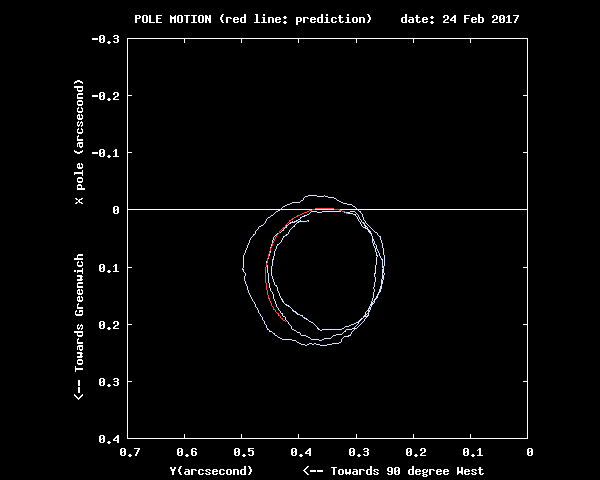Figure 1: Polar motion since 2006 with 180 day prediction in Yellow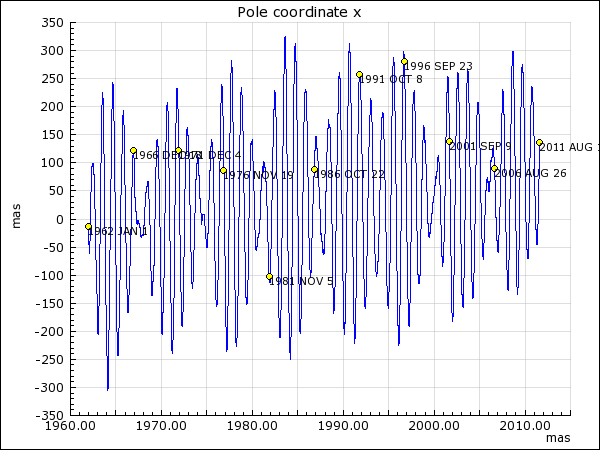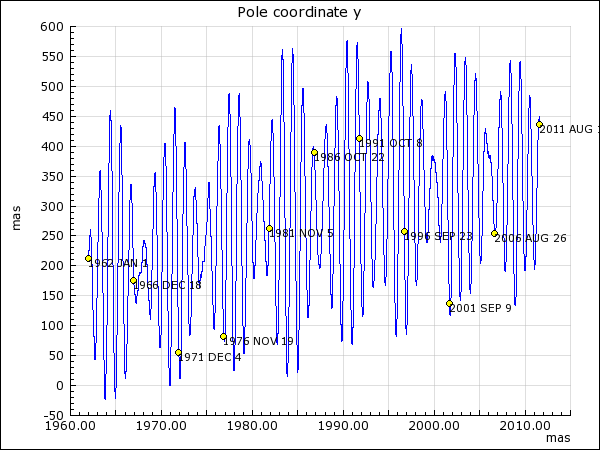Figure 2: x and y pole coordinates from 1962 to 2011 : x (left) and y (right) components The Figure 3 shows a decomposition of the x and y coordinates of the pole since 1890 into a trend, the seasonal and Chandler terms. The series shown is EOP(IERS) C 01. The residual motion in the lower part of the figures includes irregularities with recurrence times ranging from days to years that are forced by the atmosphere. Polar motion has diurnal and semi-diurnal variations with amplitudes of a fraction of millisesond of arc (mas) that are due to the oceanic tides. A correction model is available in the IERS Conventions (2003). A Fortran function for computing this effect is available on our WEB site.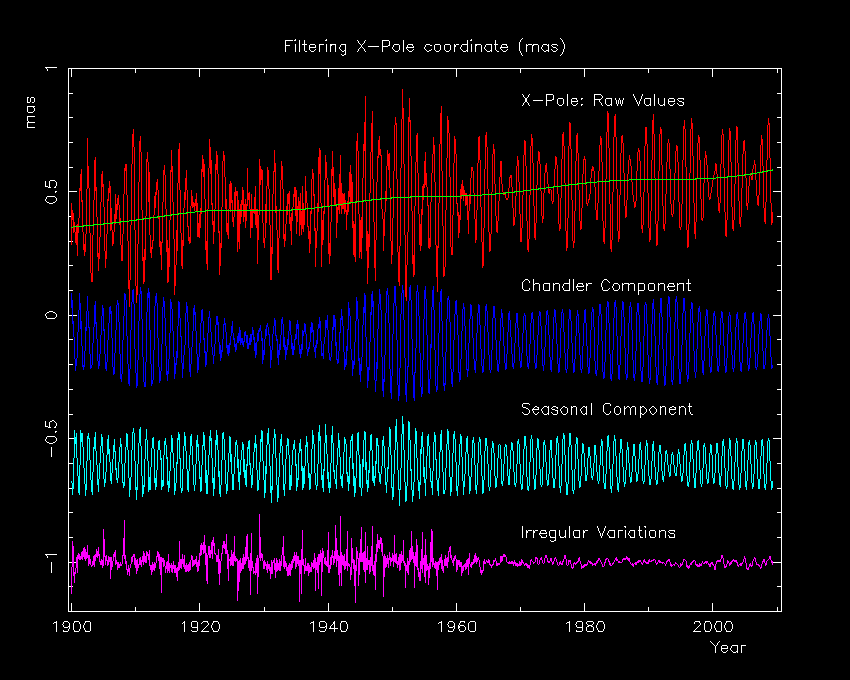Figure 3: Decomposition of x (left) and y (right) pole coordinates since 1890 in trend, Chandler term and seasonal termFigure 4 : Filtered pole. This Table or this give the smoothed polar motion in the IERS Terrestrial Reference Frame, obtained by gaussian filtering.